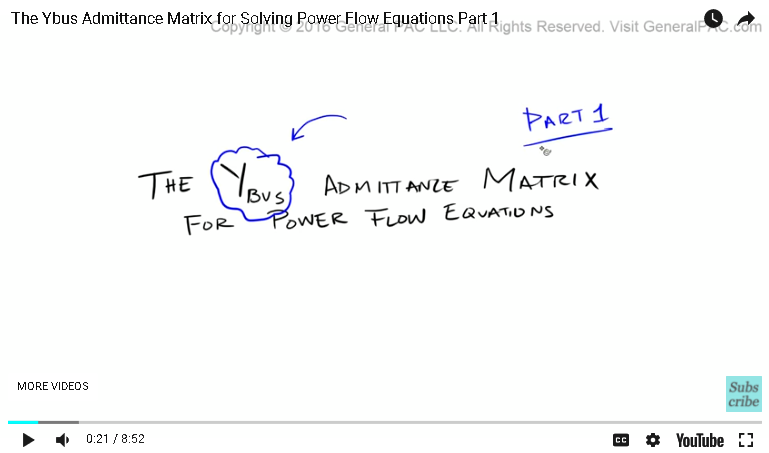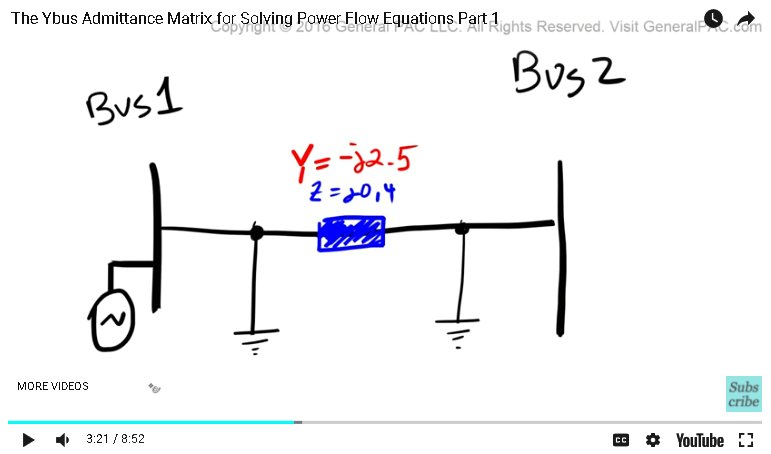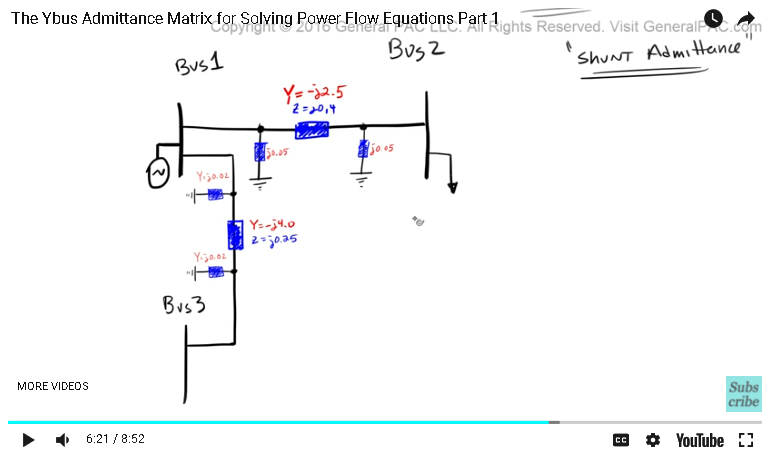This video was brought to you by GeneralPAC.com, making power systems Intuitive, Open and Free for Everyone, Everywhere. Consider subscribing and supporting through patreon.com/GeneralPAC. This is a mechanism for you to support us financially so we can continue making high quality power system video tutorials. Our corporate sponsor for this topic is AllumiaX.com from Seattle, Washington. Contact them for industrial and commercial power system studies.

In this tutorial we're going to be looking at the Ybus admittance matrix for solving power flow problems, or power flow equations. Now we're going to take a closer look at constructing the Ybus matrix and understand exactly what all of the elements actually mean from an intuitive perspective. This is part one.The Ybus admittance matrix is best understood through an example. So we're going to set up for an example and kind of talk through this entire process. So suppose that we have bus one, and we'll label it bus one right here. Now, this bus one, let just say that bus one had some sort of generator that was connected to it. And there was a transmission line that connected bus one to bus two. So let me draw this a little bit better. So the transmission line that connects bus one to bus two has some sort of impedance to it. And impedance is modeled by Z. Now impedance is equal to there's a resistive component of an impedance, plus there's a reactance component of an impedance. So we're going to model this impedance by this square box. So let's color that in.

So let's just say that Z is equal to J 0.4. We're saying that there's no resistive component here. So there's no resistive component, it's all reactive component. And keep in mind that this is just an example. So the admittance of this transmission line is equal to Y, right? And Y is equal to one over Z. So this is the admittance, so that's the admittance, and this is the impedance. So the admittance is equal to one over Z. That is equal to one over J 0.4. And if you calculate that, that is equal to negative J 2.5.

Now if you're having a hard time understanding these equations, that's all right. There's another video that was done that really got into the actual power flow equations. And a link to this particular tutorial, where we talk about all of these terminologies and can be found at the bottom of this particular video. Because admittance equals one over Z, the admittance of this transmission line then becomes Y is equal negative J 2.5. That is pretty well understood, right?

So let's clean this up a little bit. Okay. Let's make this stuff a little bit smaller. Let's also say that this transmission line was a medium length transmission line. So now this medium length transmission line also has this term called shunt admittance. And all this means is that since this is a medium length transmission line, we have to consider the shunt admittance of this line. And which we will by simply, let me just zoom in here. So there's a shunt admittance at two points, on the left side and the other on the right side. So, we have to model this shunt admittance, which we will by this yellow box, or rectangle. Let's say that the value of this shunt admittance for this particular branch was equal to J 0.05 and the same thing over here, J 0., make this guy a little bit smaller. Okay, and we're now going to zoom back out.Okay, so this is looking pretty good. So let's add another bus. So let's say that we had bus number three. And there was a transmission line that connected bus one to bus three. So let's draw that over here, and for the sake of aesthetics, let's just clean this up a little bit. Let's just move this here and move that there. So now, just like the impedance that was given for the transmission line between bus one and bus two, there's also an impedance that's given between the transmission line for bus one and bus three. So let's model that here.

Let's say that the impedance that was given, that impedance is equal to 0.25. Now because impedance is equal to 0.25, we now that admittance is going to equal one over the impedance and that's going to equal one over J 0.25, and we know clearly that that is negative J 4.0. we know that Y, which is the admittance, is equal to negative J 4.0. So let's now clean this up a little bit.

So just like this particular transmission line, let just assume that the transmission line that spans bus one and bus three was also a medium length transmission line. So let's model the shunt admittance for this transmission line. So we're going to zoom in, we're going to call this particular admittance at this line is equal to J 0., so let's zoom in. We're going to say that this is equal to J 0.02. And then we're just simply going to copy that admittance on the other end. And that admittance is also this valley, J 0.02.

So, we're looking pretty good now. And let, let just say the bus two has some sort of load that was associated to it, and then same thing with bus three. This will represent our load. And the last thing is, is that we're going to assign some voltage values here. So this the magnitude of voltage one and this is the angle of voltage one, and this is a tilda. So similarly, we're going to have the magnitude of voltage two defined like that, and then tilda two is the angle of the voltage. And then same thing over here.And then to complete part one, we're just going to write out our matrix. So Ybus, that's going to equal, so Y one/one represents the admittance for bus one, Y one/two represents the admittance between bus one and bus two, Y one/three represents the admittance between bus one and bus three. Similarly, Y two/one represents the admittance between bus two and bus one, Y two/two is the admittance for bus two, Y two/three is the admittance between bus two and three. And as you can see, bus two and three doesn't have any admittance associated to it, so that value is going to equal zero. But we'll cover that in part two. And then Y three/one, Y three/two and Y three/three.

So this entire thing is a matrix, okay? So this video now concludes part one. This video was brought to you by generalpac.com, making power systems intuitive. Visit this website for more power system tutorials. Now if you have any questions or comments, please do so by clicking on the forum link that's found at the bottom of this screen. The forum is a platform to ask more detailed questions and have the community respond to those questions. So if you have any questions about this stuff, click on the link to the forum and ask away.

Now if you haven't already, please go ahead and click on the bottom right corner of this screen, there should be a subscribe button. Subscribe to this channel to be connected to more power systems video tutorials. Thank you.

#### Greetings from the GeneralPAC Team!

We make high-quality Power Systems Video Tutorials on complex topics that are free and open to everyone!  Thank you so much for supporting us through Patreon so we can continue doing good and valuable work.

What is Patreon and why do we use it?

Patreon is a fantastic portal that allows our fans and community to make monthly contribution (like Netflix subscription) so we can continue creating high-quality power systems video tutorials. In return, you get access to incredible perks like voting on future topics, getting your questions answered, access to VIP Q/A webinars with the creators of GeneralPAC, and much more! We THANK YOU for supporting us

Why do we need your support?

An incredible amount of time and effort is needed to develop high-quality video tutorials. Each video (Part 1 for example) takes approximately 10 hours to complete which includes learning the concept ourselves, brainstorming creative ways to teach and explain the concepts, writing the script, audio recording, video recording, and editing. It's no wonder why Hundreds-of-Thousands of people have watched, liked, subscribed, and left positive comments on Youtube channel. Your support truly makes all the difference.